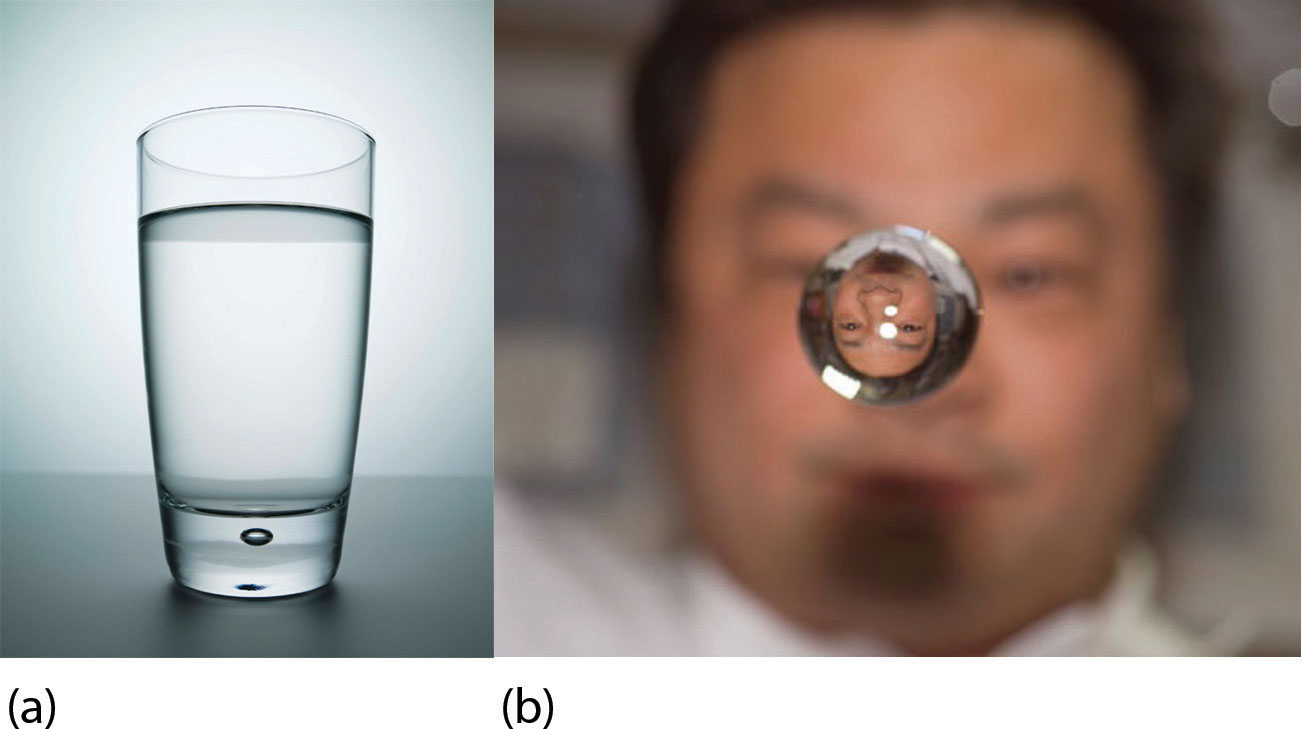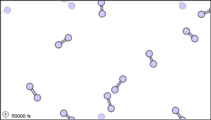# 9.3: Phase Transitions

•• Anonymous
• LibreTexts
$$\newcommand{\vecs}{\overset { \rightharpoonup} {\mathbf{#1}} }$$ $$\newcommand{\vecd}{\overset{-\!-\!\rightharpoonup}{\vphantom{a}\smash {#1}}}$$$$\newcommand{\id}{\mathrm{id}}$$ $$\newcommand{\Span}{\mathrm{span}}$$ $$\newcommand{\kernel}{\mathrm{null}\,}$$ $$\newcommand{\range}{\mathrm{range}\,}$$ $$\newcommand{\RealPart}{\mathrm{Re}}$$ $$\newcommand{\ImaginaryPart}{\mathrm{Im}}$$ $$\newcommand{\Argument}{\mathrm{Arg}}$$ $$\newcommand{\norm}{\| #1 \|}$$ $$\newcommand{\inner}{\langle #1, #2 \rangle}$$ $$\newcommand{\Span}{\mathrm{span}}$$ $$\newcommand{\id}{\mathrm{id}}$$ $$\newcommand{\Span}{\mathrm{span}}$$ $$\newcommand{\kernel}{\mathrm{null}\,}$$ $$\newcommand{\range}{\mathrm{range}\,}$$ $$\newcommand{\RealPart}{\mathrm{Re}}$$ $$\newcommand{\ImaginaryPart}{\mathrm{Im}}$$ $$\newcommand{\Argument}{\mathrm{Arg}}$$ $$\newcommand{\norm}{\| #1 \|}$$ $$\newcommand{\inner}{\langle #1, #2 \rangle}$$ $$\newcommand{\Span}{\mathrm{span}}$$$$\newcommand{\AA}{\unicode[.8,0]{x212B}}$$

##### Learning Objective
• Describe what happens during a phase change.

Substances can change phase—often because of a temperature change. At low temperatures, most substances are solid; as the temperature increases, they become liquid; at higher temperatures still, they become gaseous.

The process of a solid becoming a liquid is called melting. The opposite process, a liquid becoming a solid, is called freezing. For any pure substance, the temperature at which melting occurs—known as the melting point—is a characteristic of that substance. It requires energy for a solid to melt into a liquid. Generally, the temperature at which something freezes is the same as its melting point. But melting point is how it is generally tabulated. During melting, energy goes exclusively to changing the phase of a substance; it does not go into changing the temperature of a substance. Hence melting is an isothermal process because a substance stays at the same temperature. Only when all of a substance is melted does additional energy go to changing its temperature.

What happens when a solid becomes a liquid? In a solid, individual particles are stuck in place because the intermolecular forces cannot be overcome by the energy of the particles. When more energy is supplied (e.g., by raising the temperature), there comes a point at which the particles have enough energy to move around, but not enough energy to separate. This is the liquid phase: particles are still in contact, but are able to move around each other. This explains why liquids can assume the shape of their containers: the particles move around and, under the influence of gravity, fill the lowest volume possible (unless the liquid is in a zero-gravity environment—see Figure $$\PageIndex{1}$$.Figure $$\PageIndex{1}$$: Liquids and Gravity. (a) A liquid fills the bottom of its container as it is drawn downward by gravity and the particles slide over each other. (b) A liquid floats in a zero-gravity environment. The particles still slide over each other because they are in the liquid phase, but now there is no gravity to pull them down. Source: Photo on the left © Thinkstock. Photo on the right courtesy of NASA, http://www.nasa.gov/mission_pages/st...image_009.html.

The phase change between a liquid and a gas has some similarities to the phase change between a solid and a liquid. At a certain temperature, the particles in a liquid have enough energy to become a gas. The process of a liquid becoming a gas is called boiling (or vaporization), while the process of a gas becoming a liquid is called condensation. However, unlike the solid/liquid conversion process, the liquid/gas conversion process is noticeably affected by the surrounding pressure on the liquid because gases are strongly affected by pressure. This means that the temperature at which a liquid becomes a gas, the boiling point, can change with surrounding pressure. In fact, it is possible for this phase change to occur at temperatures below the boiling point through a process called evaporation.

What happens when a liquid becomes a gas? We have already established that a liquid is composed of particles in contact with each other. When a liquid becomes a gas, the particles separate from each other, with each particle going its own way in space. This is how gases tend to fill their containers. Indeed, in the gas phase most of the volume is empty space; only about 1/1,000th of the volume is actually taken up by matter (Figure $$\PageIndex{1}$$). It is this property of gases that explains why they can be compressed, a fact that is considered in a previous chapter.Figure $$\PageIndex{2}$$: Sub-microscopic view of the diatomic molecules of the element bromine (a) in the gaseous state (above 58°C); (b) in liquid form (between -7.2 and 58.8°C); and (c) in solid form (below -7.2°C). As a solid, the molecules are fixed, but fluctuate. As a liquid, the molecules are in contact but are also able to move around each other. As a gas, most of the volume is actually empty space. The particles are not to scale; in reality, the dots representing the particles would be about 1/100th of the size depicted.

There is also a phase change where a solid goes directly to a gas:

$\text{solid} \rightarrow \text{gas} \label{Eq3}$

This phase change is called sublimation. Each substance has a characteristic heat of sublimation associated with this process. For example, the heat of sublimation (ΔHsub) of H2O is 620 cal/g.

Chemical equations can be used to represent a phase change. In such cases, it is crucial to use phase labels on the substances. For example, the chemical equation for the melting of ice to make liquid water is as follows:

$H_2O(s) → H_2O(ℓ)\nonumber$

No chemical change is taking place; however, a physical change is taking place.

The details of these phase changes are outlined within the subsections of this section. Phase changes between solids and other physical states are discussed in 9.3.1. Phase changes between liquids and gases are discussed in 9.3.2. Calculations for any of these phase changes are discussed in 9.3.3. And finally, we will look at how to graphically show the relationship between energy transfer and changes in physical state and temperature in 9.3.4.

## Summary

• Phase changes can occur between any two phases of matter.
• All phase changes occur with a simultaneous change in energy.
• All phase changes are isothermal.

This page titled 9.3: Phase Transitions is shared under a mixed license and was authored, remixed, and/or curated by Anonymous.